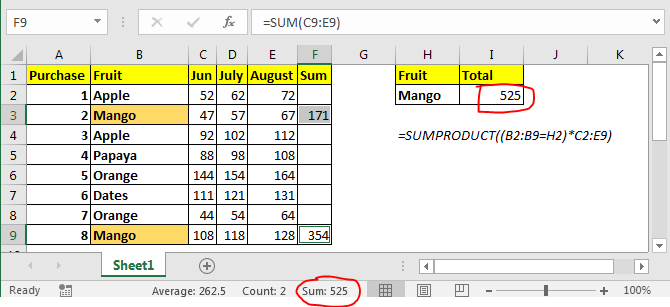# How to Sum Multiple Columns with Condition

We know how to sum values from one column on multiple condition. We use SUMIFS function for that. But how do we sum multiple columns on one condition. This article is all about summing values from multiple columns on condition.We can sum multiple columns on one condition without using SUMIF function. Here we will use SUMPRODUCT function of excel.
Generic Formula

=SUMPRODUCT((criteria_range=criteria)*(sum_range))

Criteria_range: This is the range in which criteria will be matched.
Criteria: this is the criteria or condition.
Sum_range: the sum range. This can have multiple columns but same rows as criteria range.

Let’s see it in action.
Example: Sum the money spent on mango.

In above image, we have this table of amount spent on different fruits in different months. We just need to get the total amount spent on mangoes in all these months.

In I2 the formula is

=SUMPRODUCT((B2:B9=H2)*C2:E9)

This returns 525 as total amount spent on mangos. You can see this in image above.
How it works?
Well, it is easy. Let’s break down the formula and understand it in peaces.
(B2:B9=H2): This part compares each value in range B2:B9 with H2 and returns an array of TRUE and FALSE. {FALSE;TRUE;FALSE;FALSE;FALSE;FALSE;FALSE;TRUE}.

(B2:B9=H2)*C2:E9: Here we multiplied each value in above array with values in C2:E9. C2:C9 are also treated as an 2D array. Finally this statement returns a 2D array of {0,0,0;47,57,67;0,0,0;0,0,0;0,0,0;0,0,0;0,0,0;108,118,128}.
Now SUMPRODUCT looks like this:
SUMPRODUCT({0,0,0;47,57,67;0,0,0;0,0,0;0,0,0;0,0,0;0,0,0;108,118,128}). It has values of mango only. It sums them up and returns the result as 525.

Another method can be having a totals column and then use it with SUMIF function to get the sum of all columns. But that’s not what we want to do.

So yeah guys, this how you can sum multiple columns with condition without using sumif function. Let me know if you have any doubts regarding this article or any other article on this site. You can also ask queries regarding Excel 2019, 2016, 2013 and older.

Terms and Conditions of use

The applications/code on this site are distributed as is and without warranties or liability. In no event shall the owner of the copyrights, or the authors of the applications/code be liable for any loss of profit, any problems or any damage resulting from the use or evaluation of the applications/code.